RMFTL TRANSFORM ALGORITHM CHARACTERISTICS

(From RMFTL Reference Manual, DOS version)

Two significant advantages afforded by the RMFTL multidimensional transform routines and their underlying algorithm are the reduced number of complex multiplications required to evaluate transforms relative to other methods, and the minimal number of read/write accesses required when the transform array is stored externally.

Here, for comparison with other methods, an estimate of the number of complex multiplications and an exact expression for the number of external read/write accesses required by the RMFTL transform routines are given.

COMPLEX MULTIPLICATIONS

To simplify the comparison of RMFTL with other FFT methods, consider the special case of the transform of a complex hypercubic array of dimensionality D.   By "hypercubic" it is meant that the array has the same extent in all D directions.   Let the extent along any edge be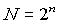.

The approximate number of complex multiplications required to evaluate a one-dimensional FFT of extent N by the Cooley-Tukey or Sande-Tukey methods is (1)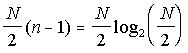.

Available multidimensional FFT implementations simply apply the one-dimensional algorithm, in one form or another, iteratively to build up the multidimensional transform.   In the example being considered, there are D orthogonal directions within the array and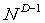"rows" of extent N parallel to each.   Consequently, the D-dimensional transform can be evaluated by forming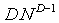one-dimensional transforms of extent N.   The approximate number of complex multiplications required is therefore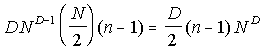.

To the same degree of approximation, the number of complex multiplications required by the RMFTL multidimensional algorithm to evaluate the same D-dimensional hypercubic transform is(2)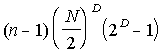.

The ratio of the RMFTL to the "conventional" approximations is,

where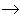indicates the behavior for large D.

Note that the relative performance of the RMFTL transform algorithm is independent, in this approximation, of the size of the array and that it improves with increasing dimensionality.   Fewer multiplications mean decreased execution time and, generally, less roundoff error in the final result.

Below is a short table of the relative number of complex multiplications required by the RMFTL transform algorithm as a function of dimensionality D derived from the above expression.

 D RMFTL 1 1.000 2 0.750 3 0.583 4 0.469 5 0.388 6 0.328 7 0.283 8 0.249

EXTERNAL DATA ACCESSES

When the array to be transformed exceeds the capacity of high speed main memory, it must be stored and manipulated from an "external" memory device such as a disk or magnetic tape.   Such devices, being electromechanical in nature, are inherently much slower than main memory.   The time required to evaluate a transform becomes critically dependent upon, and could easily be dominated by, the time required to access data records from external memory.

For the purposes of this discussion, the "row" direction is defined to correspond to the sequence of data in an external record.   That is, an external data record and a "row" of the array to be transformed are, by definition, synonymous.   As used here, an "access" is defined to be one complete read/write cycle; that is, the reading of an external record and the subsequent writing back to external memory of the, presumably, modified data record.

The development of the multidimensional transform of an external data array presents a special problem with regard to transformations other than those along the row direction because the data cannot be read "across" records.   For example, there is no easy way to read "columns" of data from a magnetic tape whose records contain the "rows" of a data array.   The process of reading rows to build up a few "columns" in main memory, transforming them, merging and writing them back as rows to external memory, and repeating the process until all "columns" in directions normal to the rows have been transformed can be extremely, if not prohibitively, time consuming.

Many ad hoc schemes have been devised to deal with this problem, but all make inefficient use of the data available each time a record is read.   None would appear to be a useful standard with respect to which to compare RMFTL.

On the other hand, the method due to Brenner, (3) because it, like RMFTL, makes efficient use of row data, appears to be inherently more efficient than any of the ad hoc schemes and so will be used for comparison purposes with RMFTL.

Consider the transform of a D-dimensional complex data array with extents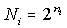(i = 1,2,3 ... D) stored on external memory.   The Brenner method, when implemented for external data, requires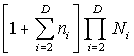accesses if the transform is taken along all D directions.   If the transform is suppressed along the row direction, the "1" in the brackets should be omitted.   If the transform is suppressed along the j-th direction, where j = 2,3,4 ... D, the term of the summation corresponding to i = j should be omitted.

The number of accesses required by the RMFTL routines ("X" type) to evaluate the same complex transform is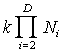where k has the value 1 if the transform is taken only along the row direction.(4)   Otherwise, whether the transform is or is not taken along the row direction, k is equal to the greatest of the ni for i = 2,3,4 ... D, excluding those corresponding to (non-row) directions along which the transform is suppressed.

Clearly, the Brenner method does not achieve the efficiency of the RMFTL algorithm, in terms of the number of external data accesses.

To make the comparison somewhat more obvious, consider the special case of equal extentsin all D directions, with the transform taken along all directions.   The number of accesses required by the Brenner method becomes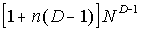,

and the number required by RMFTL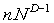.

The ratio of the number of RMFTL accesses to the number required by the Brenner method is then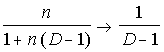whereindicates the behavior for large n.

The efficiency, in this case, of the RMFTL transform algorithm relative to the Brenner method, in terms of external data accesses, increases with increasing dimensionality.   Since the Brenner method is itself much more efficient than most ad hoc schemes, the efficiency of RMFTL relative to other implementations that process external data is likely to be considerably greater than that suggested by the comparison with the Brenner method.

 1 To simplify the resulting expressions, estimates here neglect the savings of multiplications afforded by several well known techniques.   For example, the handling of ± 1, ± i and 1 ± i as special cases that do not require actual multiplication operations, as well as the "passing through" and combining of multiplications from several stages of processing.   The relative savings of multiplications afforded by the RMFTL multidimensional algorithm, an estimate of which is given here, are distinct from these techniques, which are routinely employed in available one-dimensional FFT implementations and are utilized as well in the RMFTL multidimensional transforms.   Moreover, since the objective here is to estimate the relative improvement afforded by RMFTL, the omission of corrections for these techniques tends to cancel in the final estimate. 2 If the transform is suppressed along k of the D directions, where k = 0,1,2, ... D, the approximate number of multiplications required by the RMFTL transform algorithm generalizes to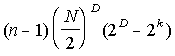3 See Numerical Recipes, Wm. H. Press et al., Cambridge University Press, §12.2 and §12.11 (1986). 4 This expression holds also for the RMFTL real transforms of external data, except that k takes the value 2 if the transform is taken along the row direction only.